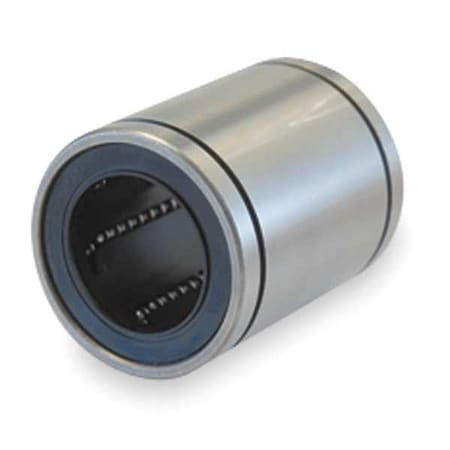Dayton

# Ball Bushing Bearing, Closed, Bore 5/8 InTap to zoom
Hover or click to zoom
\$28.38
• Item: Linear Ball Bearing
• Type: Closed
• Bore Dia.: 0.625 in
See full product details
Orders over \$75 ship free.

Total: \$28.38

## Dayton #2CNK2 Specifications

• Item: Linear Ball Bearing
• Type: Closed
• Bore Dia.: 0.625 in
• Outside Dia.: 1.125 in
• Material: Stainless Steel/Polymer Cage
• Dynamic Load Capacity (Lb.): -
• Dimension C (In.): -
• Dimension JB: -
• Dimension V (In.): -
• Temp. Range (F): 0 - 176
• Dimension F (In.): -
• Dimension H (In.): -
• Dimension N Dia. (In.): -
• Mounting Hole (In.): -
• Dimension d: -
• Length (In.): 1.5 in
• Between Grooves (In.): 1.108/1.092
• Dimension E1 (In.): -
• Dimension D1: 1.059 in
• Outside Dia. Tolerance: +/-0.00050
• Dimension C1: -
• Inside Dia. Tolerance: +/-.0004
• Required Housing Bore Dia. (In.): -
• Dimension B (In.): -
• Retention Hole Location: -
• Mounting Bolt (In.): -
• Dimension W: 0.056 in
• Dimension H1: -
• Dimension YZ: -
• Dimension H3: -
• Dimension JA: -
• Dimension N2 (In.): -
• Dimension E (In.): -
• Dimension L: 1.5 in
• Dimension A1 (In.): -
• Withstand Temp. (Deg. F): -
• Includes: -
• Height: -
• Use w/Ball Bushing Number: -
• Dimension F: -
• Dimension E: -
• Width: -
• Required Housing Bore Dia. (mm): -
• Seal: -
• Slot Width (In.): -
• Retention Hole Location (In.) G: -
• Dimension H2: -
• Dimension G1 (In.): -
• Dimension L (In.): -
• Dimension D (In.): -
• Dimension J: -
• Dimension S (In.): -
• Dimension X: -
• Max. Pv: -
• Dimension A (In.): -
• Retention Hole Dia. (In.): -
• Dimension XZ: -
• Dimension Y: -
• Static Load Capacity (Lb.): 265
• Dimension C2: -
• Between Grooves (mm): -
• Agency Compliance: -
• Self-Alignment: No
• Slot Angle (Degrees): -
• Dimension H1 (In.): -
• Shaft Dia. (In.): -
• Dimension HB: -
• Application: -
• Dimension C: -
• Dimension F1 (In.): -
• For Use With: Linear Motion Shafts
• Accelerated Speed: -
• Dimension K: -
• Dimension G: -
• Dimension HA: -
• Steady State Speed: 5000 in/min
• Dimension A2 (In.): -
• Retention Hole Dia. (mm): -
• Required Hole Location: -
• Slot Width (mm): -
• Dimension T: -
• Length (mm): -
• Dimension B2: -
• Dimension G (In.): -